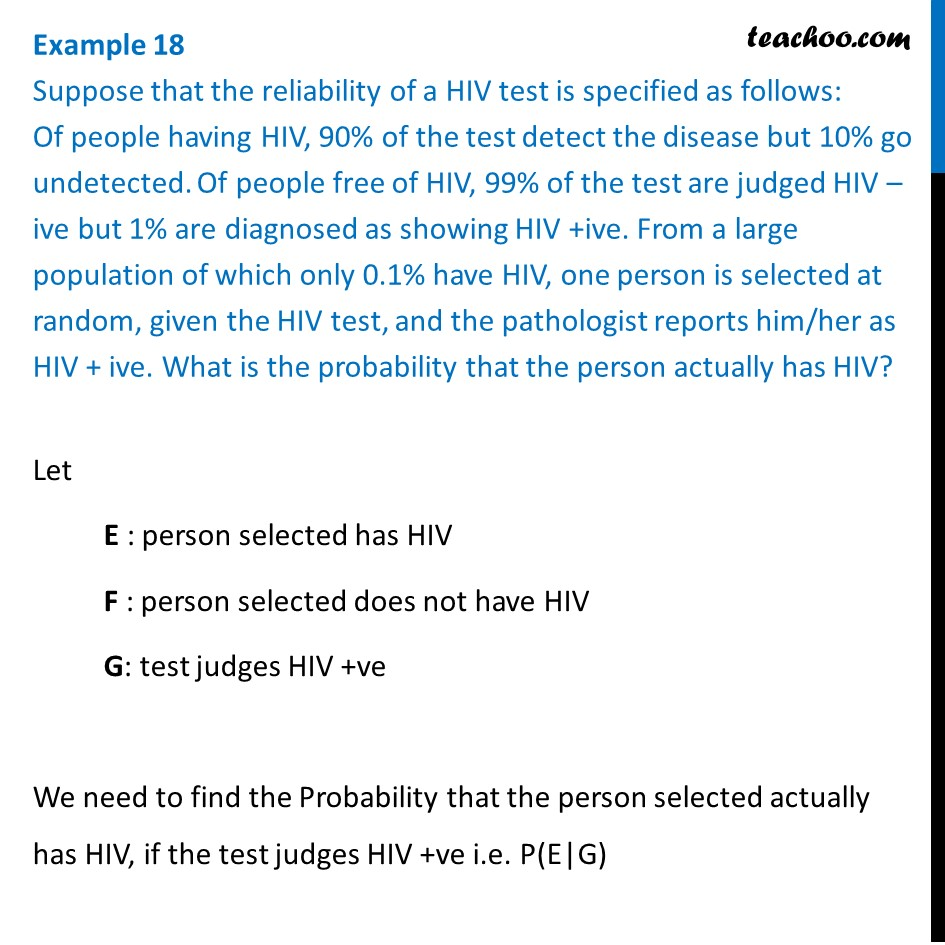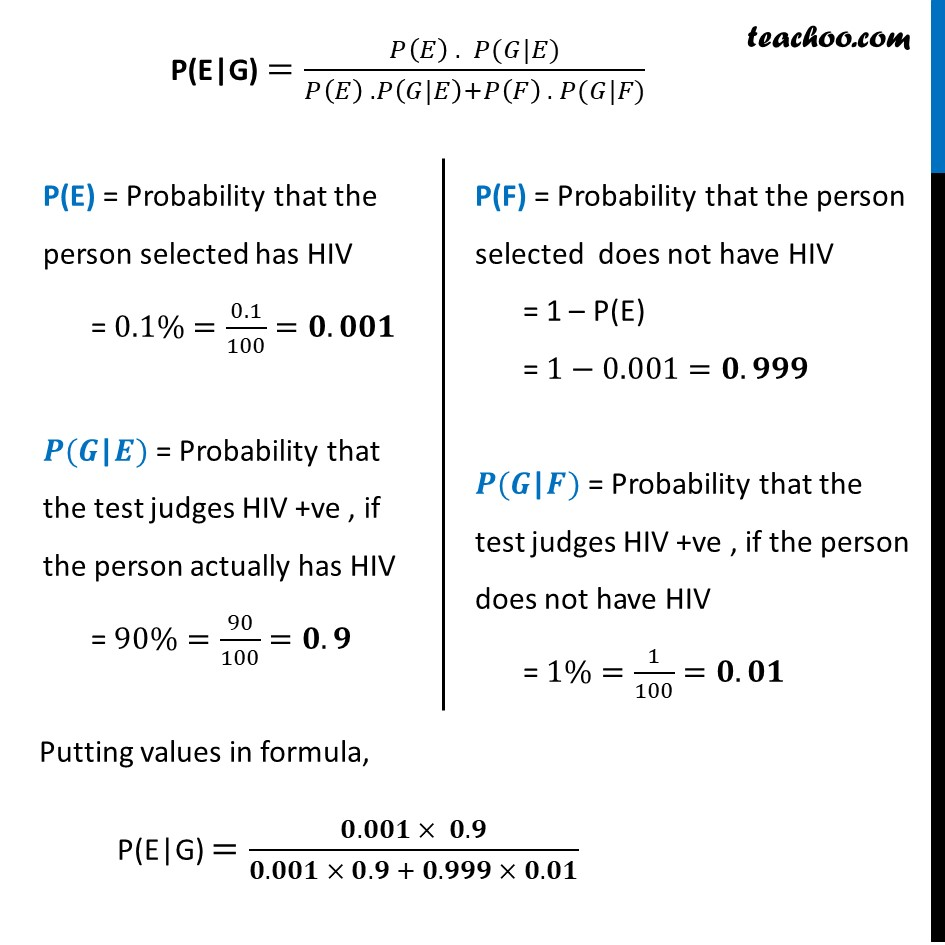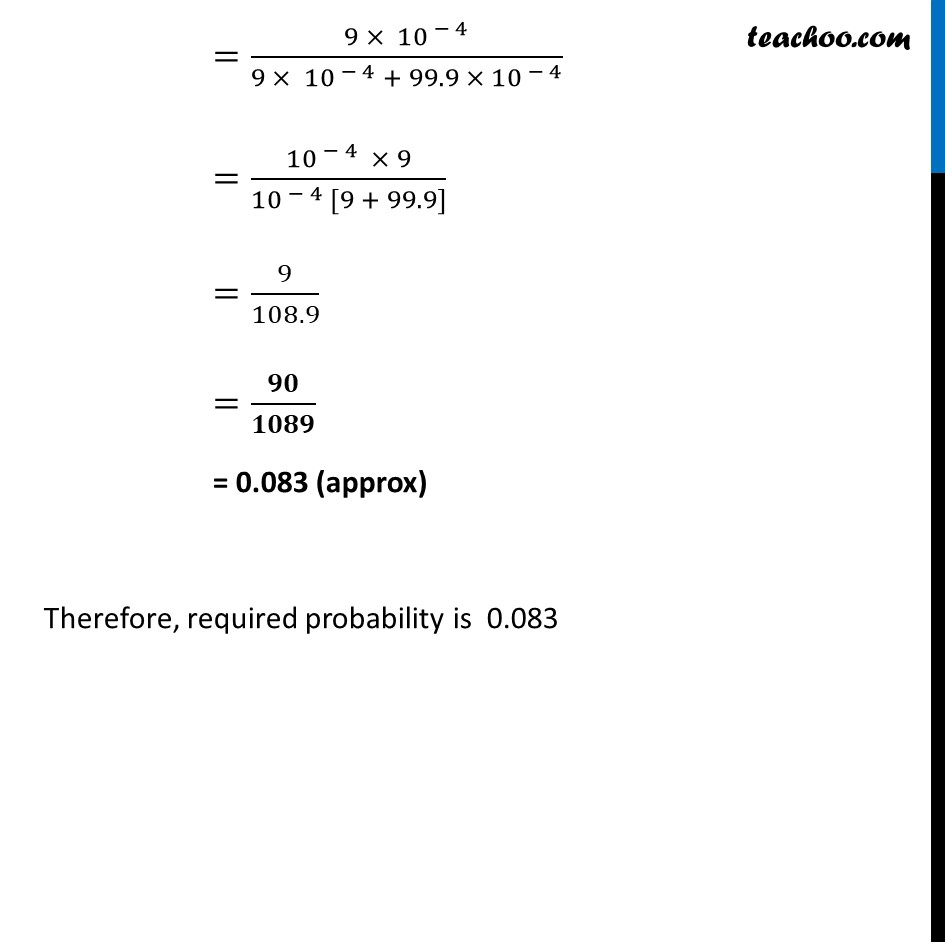Examples

Chapter 13 Class 12 Probability
Serial order wiseLearn in your speed, with individual attention - Teachoo Maths 1-on-1 Class

### Transcript

Example 18 Suppose that the reliability of a HIV test is specified as follows: Of people having HIV, 90% of the test detect the disease but 10% go undetected. Of people free of HIV, 99% of the test are judged HIV –ive but 1% are diagnosed as showing HIV +ive. From a large population of which only 0.1% have HIV, one person is selected at random, given the HIV test, and the pathologist reports him/her as HIV + ive. What is the probability that the person actually has HIV?Let E : person selected has HIV F : person selected does not have HIV G: test judges HIV +ve We need to find the Probability that the person selected actually has HIV, if the test judges HIV +ve i.e. P(E"|"G) "P(E)" = Probability that the person selected has HIV = 0.1%=0.1/100=𝟎.𝟎𝟎𝟏 𝑷(𝑮"|" 𝑬) = Probability that the test judges HIV +ve , if the person actually has HIV = 90%=90/100=𝟎.𝟗 "P(F)" = Probability that the person selected does not have HIV = 1 – "P(E)" = 1−0.001=𝟎.𝟗𝟗𝟗 𝑷(𝑮"|" 𝑭) = Probability that the test judges HIV +ve , if the person does not have HIV = 1%=1/100=𝟎.𝟎𝟏 Putting values in formula, P(E|G) =(𝟎.𝟎𝟎𝟏 × 𝟎.𝟗)/(𝟎.𝟎𝟎𝟏 × 𝟎.𝟗 + 𝟎.𝟗𝟗𝟗 × 𝟎.𝟎𝟏) =(9 × 10^( − 4))/(9 × 10^( − 4) + 99.9 ×〖 10〗^( − 4) ) =(10^( − 4) × 9)/(10^( − 4) [9 + 99.9]) =9/108.9 =𝟗𝟎/𝟏𝟎𝟖𝟗 = 0.083 (approx) Therefore, required probability is 0.083